JMSLTM Numerical Library 7.2.0
com.imsl.datamining.neural

## Interface QuasiNewtonTrainer.Error

• All Superinterfaces:
Serializable
Enclosing class:
QuasiNewtonTrainer

```public static interface QuasiNewtonTrainer.Error
extends Serializable```
Error function to be minimized by trainer. This trainer attempts to solve the problem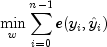where w are the weights, n is the number of training patterns,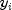is a training target output and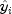is its forecast value.

This interface defines the function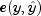and its derivative with respect to its computed value,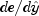.

• ### Method Summary

Methods
Modifier and Type Method and Description
`double` ```error(double[] computed, double[] expected)```
Returns the contribution to the error from a single training output target.
`double[]` ```errorGradient(double[] computed, double[] expected)```
Returns the derivative of the error function with respect to the forecast output.
• ### Method Detail

• #### error

```double error(double[] computed,
double[] expected)```
Returns the contribution to the error from a single training output target. This is the function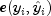.
Parameters:
`computed` - A `double` representing the computed value.
`expected` - A `double` representing the expected value.
Returns:
A `double` representing the contribution to the error from a single training output target.
```double[] errorGradient(double[] computed,
`computed` - A `double` representing the computed value.
`expected` - A `double` representing the expected value.
A `double` representing the derivative of the error function with respect to the forecast output.